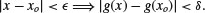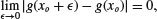B.1 CONTINUITY AND BOUNDED VARIATION

Definition: Function Function y = g(x) is a mapping from x to y.

This definition implies that for a given x, the mapping to y is unique. The inverse image x = g−1(y) may not be unique; if it is unique, then g(x) is one-to-one.

Definition: Continuous Function Function g(x) is continuous at xo if for every δ > 0, there existssuch that

(B.1)We can also write for a continuous function g(x) at xo that

(B.2)where in this case ε is positive or negative: the limit must hold approaching xo from the right and the left.

Thus, we can simply write that g(x) is continuous at xo if

(B.3)for finite g(xo). The definition in (B.1) applies to a specific point xo, meaning that a particular pairapply to that point. This can be emphasized by writing δ as a function of xo and ε:(not to be confused with the Dirac delta function defined later). For some other point ...

Get Probability, Random Variables, and Random Processes: Theory and Signal Processing Applications now with the O’Reilly learning platform.

O’Reilly members experience books, live events, courses curated by job role, and more from O’Reilly and nearly 200 top publishers.